# #326 ignore 325, only need 326 In the following exercises, consider a lamina occupying the region...

#326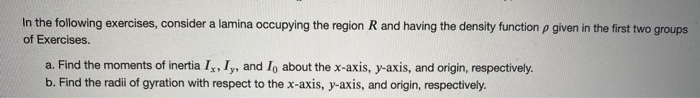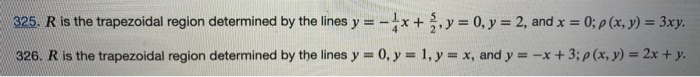ignore 325, only need 326
In the following exercises, consider a lamina occupying the region R and having the density function p given in the first two groups of Exercises. a. Find the moments of inertia IX, Iy, and I, about the x-axis, y-axis, and origin, respectively. b. Find the radii of gyration with respect to the x-axis, y-axis, and origin, respectively.
325. R is the trapezoidal region determined by the lines y=-**+ , y = 0, y = 2, and x = 0; p(x, y) = 3xy. 326. R is the trapezoidal region determined by the lines y = 0, y = 1, y = x, and y = -x +3; p(x, y) = 2x + y.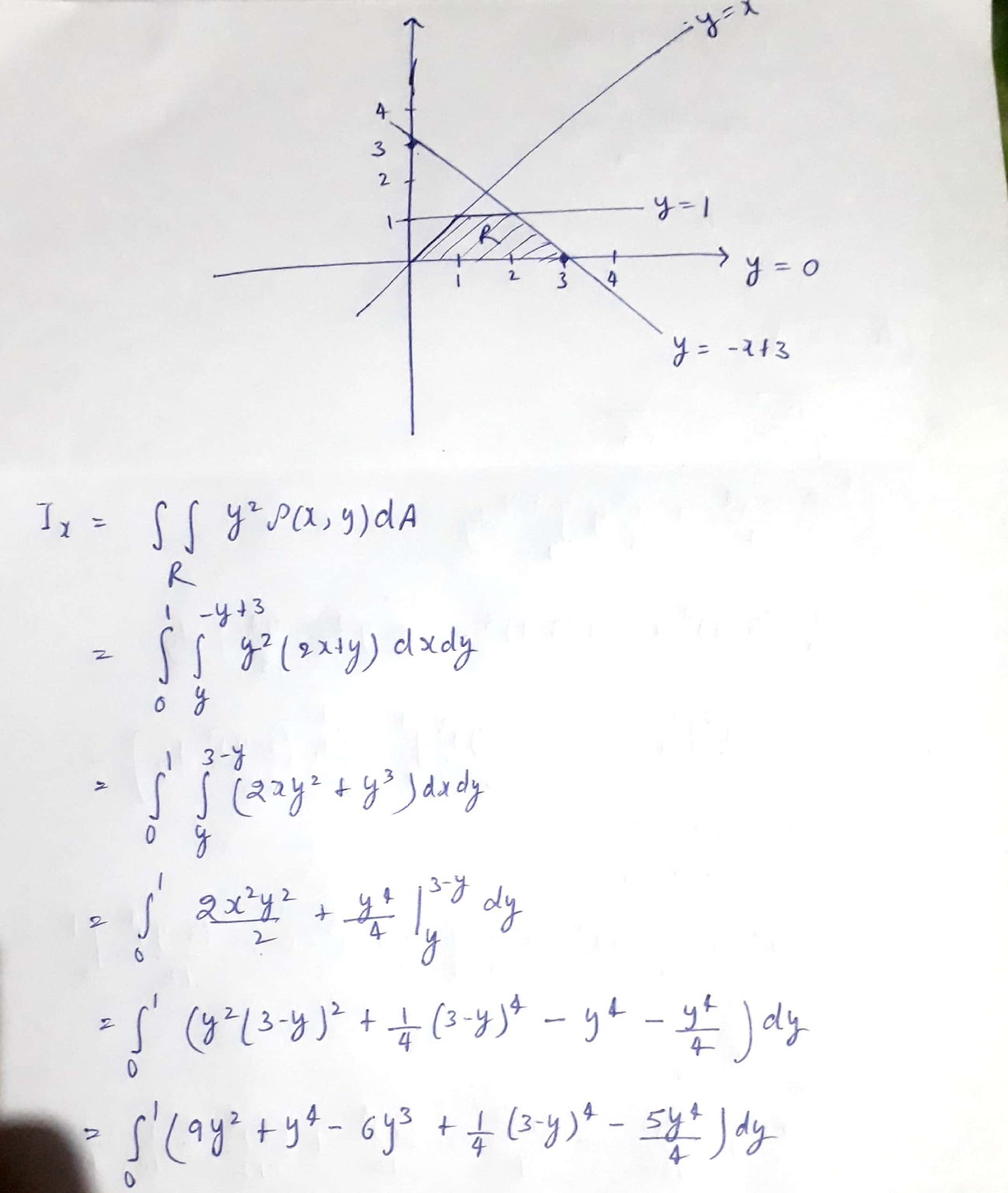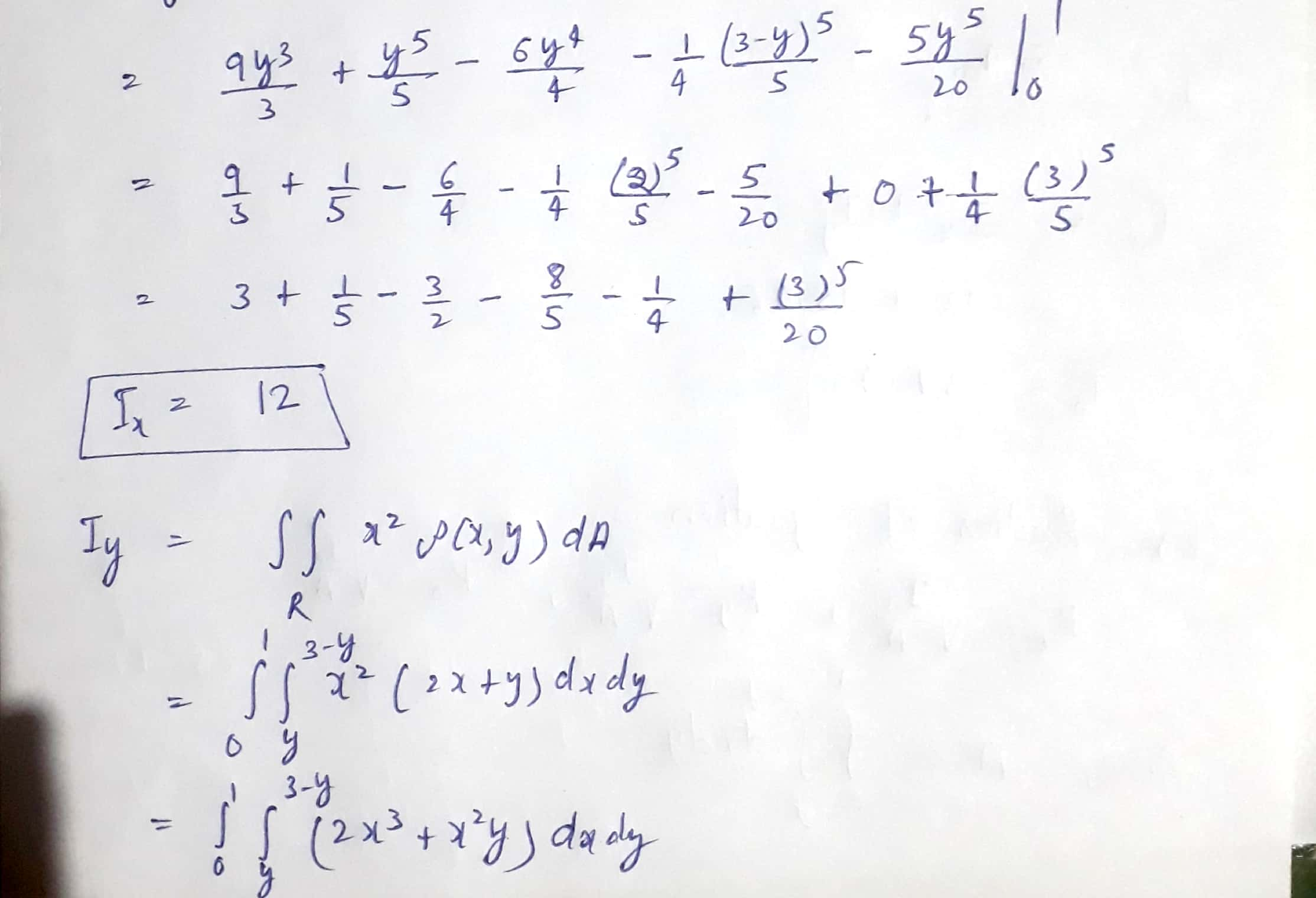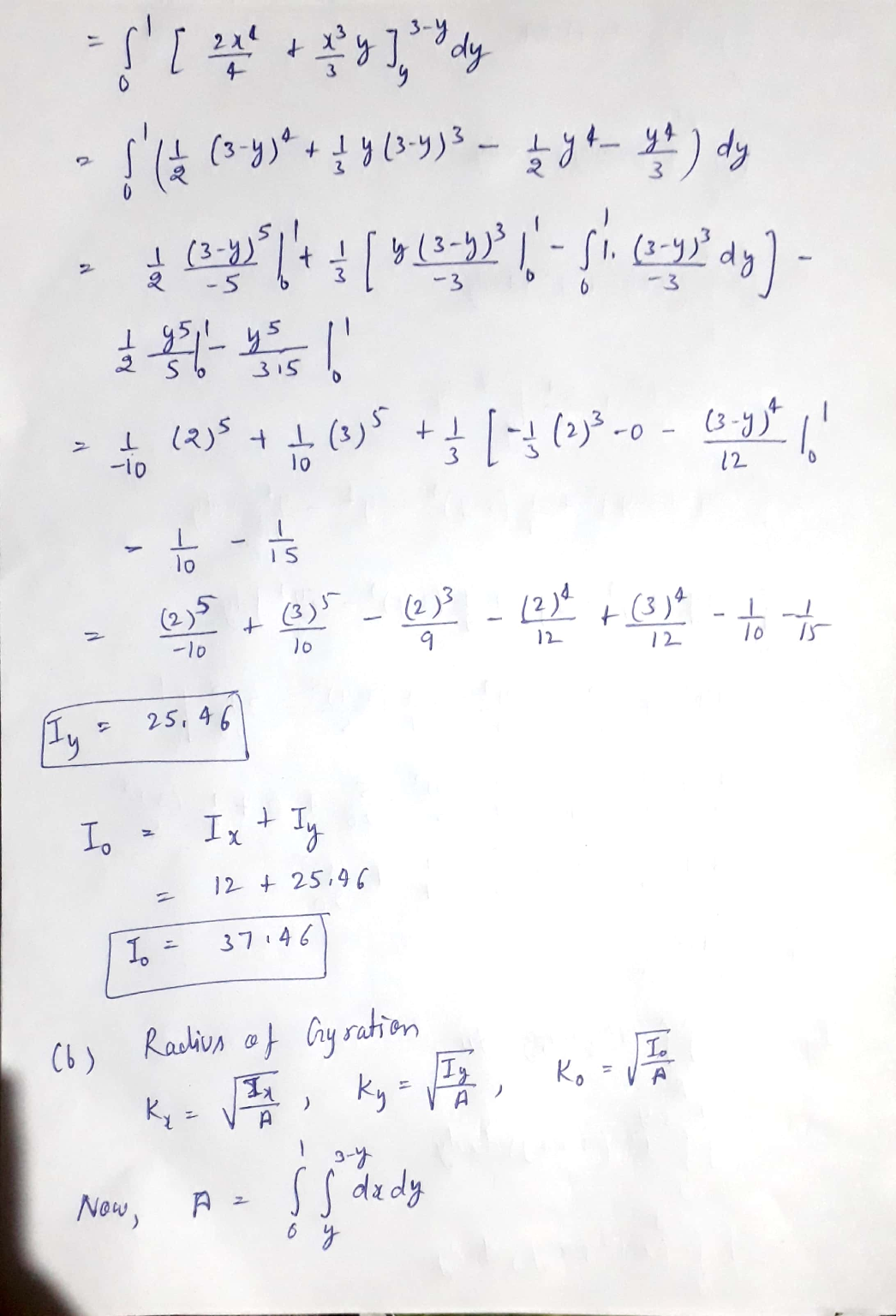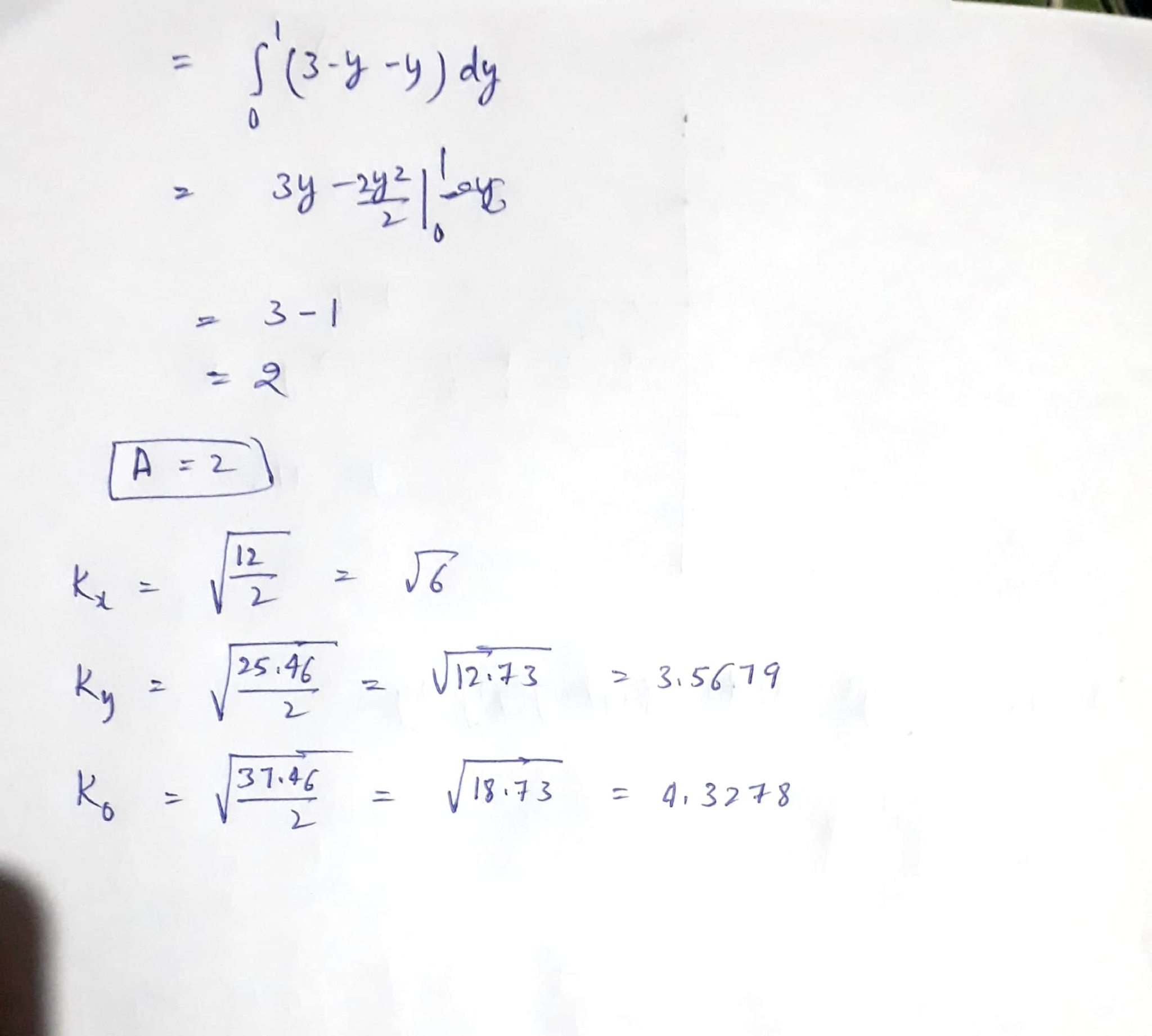##### Add Answer of: #326 ignore 325, only need 326 In the following exercises, consider a lamina occupying the region...
Similar Homework Help Questions
• ### A lamina with constant density p(x, y) = p occupies the given region. Find the moments...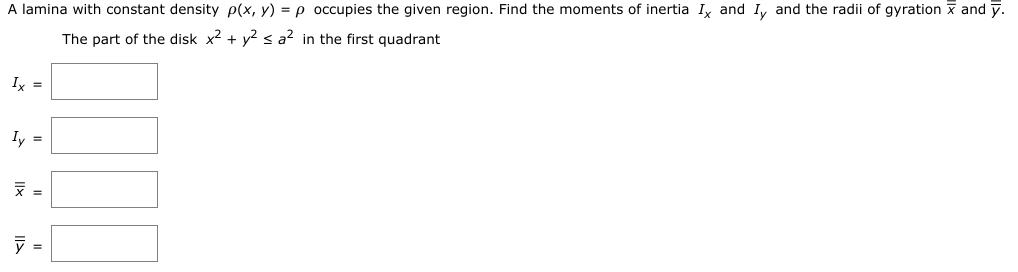A lamina with constant density p(x, y) = p occupies the given region. Find the moments of inertia Ix and ly and the radii of gyration and y. The part of the disk x2 + y2 s az in the first quadrant Ix = Iy =

• ### applications with double integrals

A lamina with constant density p(x,y)=p occupes the given region. Find the moments of inertia Ix, and Iy and the radii of gyration x and y.the triangle with vertices (0,0), (b,0), and (0,b)

• ### applications with double integrals

A lamina with constant density p(x,y)=p occupes the given region. Find the moments of inertia Ix, and Iy and the radii of gyration x and y.the region under the curve y=sinx from x=0 to x=pi

• ### applications with double integrals

A lamina with constant density p(x,y)=p occupes the given region. Find the moments of inertia Ix, and Iy and the radii of gyration x and y.the part of the disk x2+y2<=a2 in the first quadrant

• ### applications with double integrals

A lamina with constant density p(x,y)=p occupes the given region. Find the moments of inertia Ix, and Iy and the radiiof gyration x and y.The rectangle 0<=x<=b, 0<=y<=h

• ### Finding Inertia and radii of gyrationA lamina with constant density ρ(x,y)=5ρ occupies the given region. Find the moments of inertia Ix and Iy and the radii of gyration x(double hat) and y(doublehat).The triangle with vertices (0,0), (b,0), and (0,4h)Having trouble setting up the integration for Inertia, after those two are found i know the radii of gyration is for y(double hat) where10ρbh is the constant density multiplied by the area of the triangle

• ### lamina with density ρ(x,y) = 3 √{x2+y2} occupies region D, enclosed by the curve r =...

lamina with density ρ(x,y) = 3 √{x2+y2} occupies region D, enclosed by the curve r = 1−sin(θ). Which of the following statements is the best description of the center of mass of the lamina? Find the moments of intertia about the x-axis, the y-axis, and the origin for the lamina. Yes, the integrals can be done by hand, but why put yourself through that? You may round your answers to the nearest 0.01.

• ### Problem #8 : A lamina with constant density ρ(r.))-5 occupies the region under the curve y-sin(m/8) from x-0 to x-8. Find the moments of inertia 4 and Enter the values of 4 and ly (in that order) int...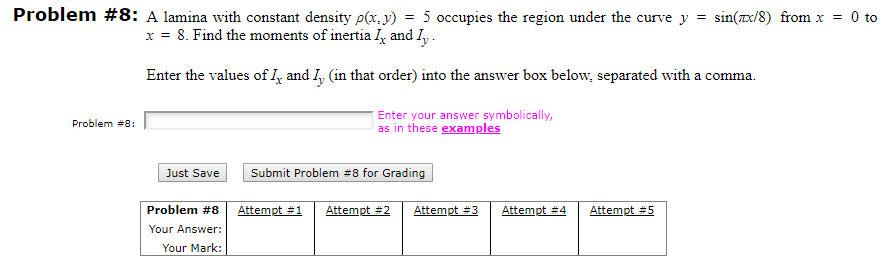Problem #8 : A lamina with constant density ρ(r.))-5 occupies the region under the curve y-sin(m/8) from x-0 to x-8. Find the moments of inertia 4 and Enter the values of 4 and ly (in that order) into the answer box below, separated with a comma. Enter your answer symbolically, as in these examples Problem #8: Just save Submit Problem #8 for Grading Problem #8 | Attempt #1 | Attempt #2 Attempt #3 Attempt #4 Attempt #5 Your Answer: Your...

• ### Hi, I need help solving number 13. Please show all the steps, thank you. :) Consider the solid Q bounded by z-2-y2;z-tx at each point Р (x, y, z) is given by mass of Q [15 pts] 9. x-4. The density Z/...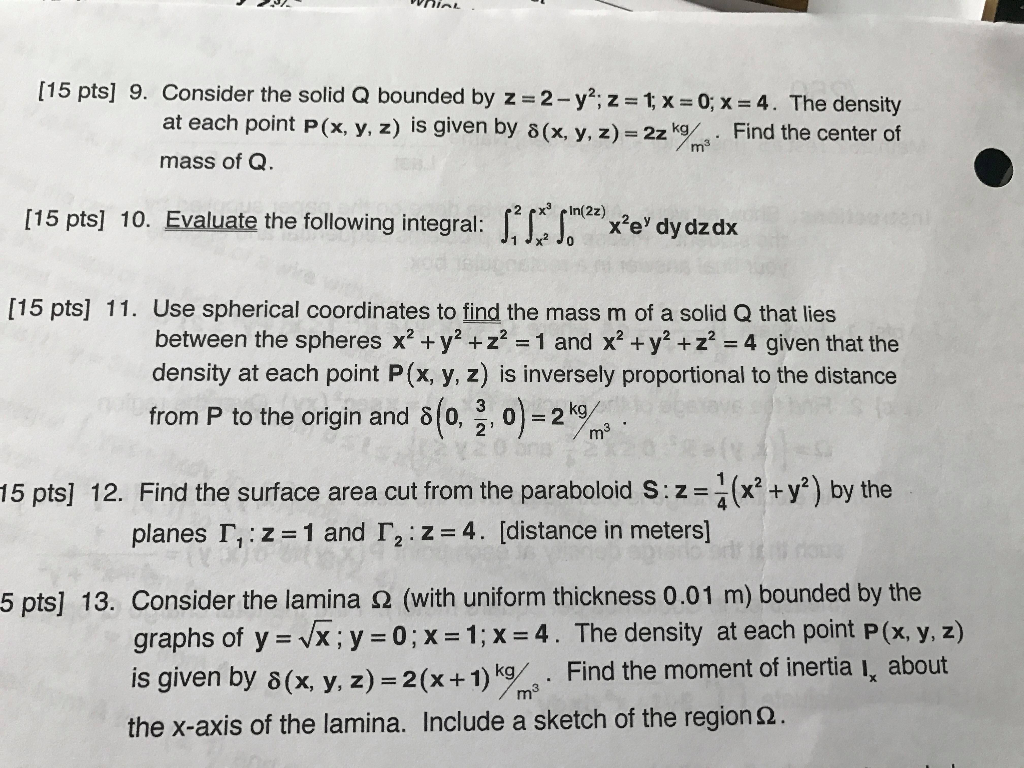Hi, I need help solving number 13. Please show all the steps, thank you. :) Consider the solid Q bounded by z-2-y2;z-tx at each point Р (x, y, z) is given by mass of Q [15 pts] 9. x-4. The density Z/m 3 . Find the center of (x, y, z) [15 pts] 10. Evaluate the following integral: ee' dy dzdx [15 pts] 11. Use spherical coordinates to find the mass m of a solid Q that lies between the...

• ### Use double integrals to licate the fentroid of a two-dimensional region. LOOK AT ALL OTHER PHOTOS...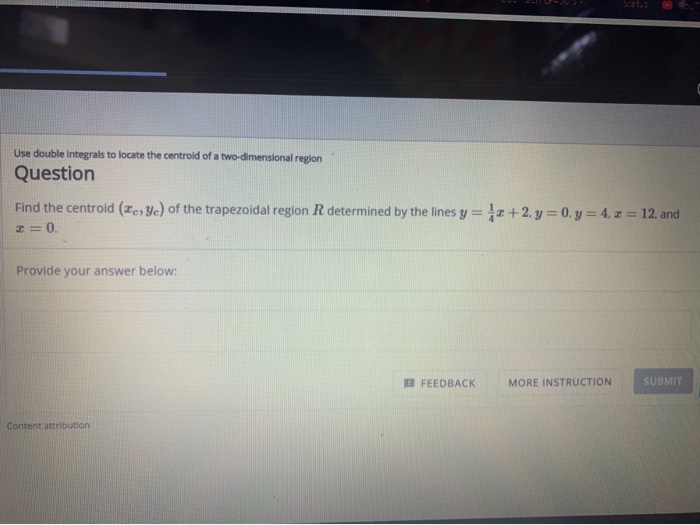Use double integrals to licate the fentroid of a two-dimensional region. LOOK AT ALL OTHER PHOTOS AS EXAMPLES AND STEPS ARE INCLUDED WITHIN!!! Use double integrals to locate the centrold of a two-dimensional region Question Find the centroid (Ic, yc) of the trapezoidal region R determined by the lines y = -x + 2 y = 0y = 4,2= 12, and =0 Provide your answer below: FEEDBACK MORE INSTRUCTION SUBMIT Content attribution Question Calculate the component of the centroid with...

Free Homework App